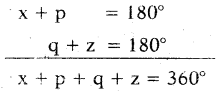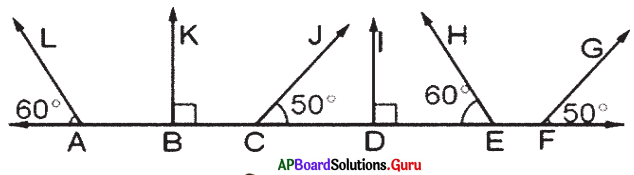SCERT AP 7th Class Maths Solutions Pdf Chapter 4 Lines and Angles Ex 4.4 Textbook Exercise Questions and Answers.

## AP State Syllabus 7th Class Maths Solutions 4th Lesson Lines and Angles Exercise 4.4

Question 1.
In the given figure, two lines p ∥ q arid r is transversal, If ∠3 = 135°, then find the remaining angles.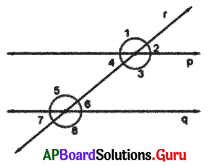Given ∠3 = 135
∠1 = ∠3 = 135° (vertically opposite angles)
∠1 = ∠5 = 135° (corresponding angles)

∠3 = ∠8 = 135° (corresponding angles)
∠3 = ∠5 = 135° (Alternate interior angles)

∠1 + ∠7 = 180° (co-exterior angles are supplementary)
135° + ∠7 = 180°
∠7 = 180°- 135° = 45°
∠6 = ∠7 = 45° (vertically opposite angles)
∠6 = ∠2 = 45° (corresponding angles)
∠4 = ∠7 = 45° (corresponding angles)
∴ ∠1 = 135°, ∠2 = 45°, ∠3 = 135°, ∠4 = 45°, ∠5 = 135°, ∠6 = 45°, ∠7 = 45°, ∠8 = 135°

Question 2.
In the given figure, $$\overleftrightarrow{\mathbf{AB}}$$ || $$\overleftrightarrow{\mathbf{CD}}$$ and $$\overleftrightarrow{\mathbf{DE}}$$ is a transversal. Find x.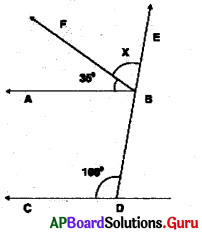Given $$\overleftrightarrow{\mathbf{AB}}$$ || $$\overleftrightarrow{\mathbf{CD}}$$ and $$\overleftrightarrow{\mathbf{DE}}$$ is a transversal line.
∠ABE = ∠CDB (corresponding angles)
∠ABF + ∠FBE = ∠CDB (we know ∠ABE = ∠ABF + ∠FBE)
From the figure ∠ABF = 35°,
∠FBE = x° and ∠CDB =100°
35° + x = 100°
⇒ 35° + x – 35° = 100° – 35°
∴ x = 65°Question 3.
In the given figure, m || n and p is transversal. Find x and y.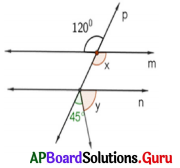Given m || n and p is transversal.
So, ∠x = 120° (vertically opposite angles)
45 + y = x (corresponding angles)
⇒ 45 + y = 120° (we know ∠x = 120°)
⇒ 45 + y – 45 = 120-45°
⇒ y = 75°
x = 120° and y = 75°

Question 4.
In the given figure, $$\overrightarrow{\mathbf{A B}}\|\overrightarrow{\mathbf{C D}}\| \overrightarrow{\mathbf{F E}}$$. Find x, y and ∠AEC.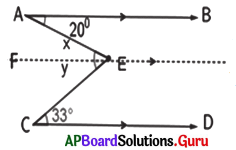Given $$\overrightarrow{\mathbf{A B}}\|\overrightarrow{\mathbf{C D}}\| \overrightarrow{\mathbf{F E}}$$
∠x = 20° (Alternate interior angles)
∠y = 33° (Alternate interior angles)
∠AEC = ∠AEF + ∠FEC
∠AEC = ∠x + ∠y
∠AEC = 20° + 33° = 53°
∴ x = 20°, y = 33° and ∠AEC = 53°

Question 5.
In the given figure, a transversal t intersects two lines p and q. Check whether p ∥q or not.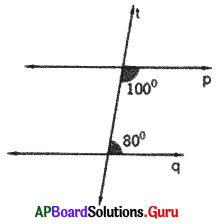If co-interior angles are supplementary, then the lines are parallel.
100° + 80° = 180° (co-interior angles supplementary)
So, p and q are parallel to each other.

Question 6.
In the given figure if l ∥m, then find x, y and z.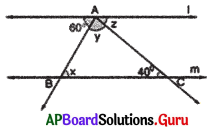Given l ∥m and AC is transversal.
z = 40° (Alternate interior angles)
l ∥m and AB is transversal. , and x + (y + z) = 180° (co-interior angles are supplementary)
x + y + z = 180°
(x + y) + 40° = 180° (co-interior angles are supplementary) (∵ z = 40°)
x + y + 40° = 180°
x + y + 40°-40° = 180° – 40°
∴ x + y = 140°
But x = y (given)
2x = 140°
∴ x = $$\frac{140^{\circ}}{2}$$ = 70° = y
So, x = 70°, y = 70° and z = 40°Question 7.
In the given figure p, q, r and s are parallel lines and t is a transversal. Find x, y and z.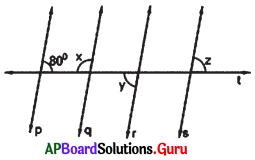p ∥ q
So, 80° + x = 180° (co-interior angles)
80° + x-80° = 180°-80°
x = 100°

q ∥ r
x + y = 180° (co-interior angles)
100° + y = 180° (we know x – 100°)
100° + y – 100° = 180°- 100°
y = 80°

r∥s
y = z (Alternate exterior angles)
y = z = 80° (we know y = 80°)
x = 100°, y = 80° and z = 80°

Question 8.
In the given figure $$\overrightarrow{\mathbf{A B}} \| \overrightarrow{\mathbf{C D}}$$ and E is a point in between them. Find x + y + z. (Hint : Draw a parallel line to $$\overrightarrow{\mathbf{A B}}$$ through E)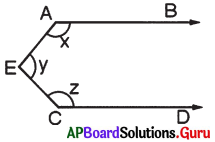Given $$\overrightarrow{\mathbf{A B}} \| \overrightarrow{\mathbf{C D}}$$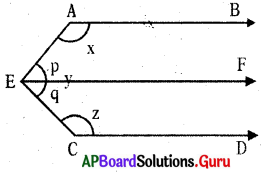Draw $$\overrightarrow{\mathrm{EF}} \| \overrightarrow{\mathrm{AB}}$$
∠BAE = x,
∠AEF = p
∠FEC = q,
∠ECD = z
∠AEC = ∠AEF + ∠FEC
y = p + q

AB ∥ EF and AE is a transversal.
∠BAE + ∠AEF = 180° (co-interior angles are supplementary)
x + p = 180° ……………….(1)
CD ∥ EF and EC is a transversal.
∠FEC + ∠ECD = 180° (co-interior angles are supplementary’)
q + z = 180° …………….(2)